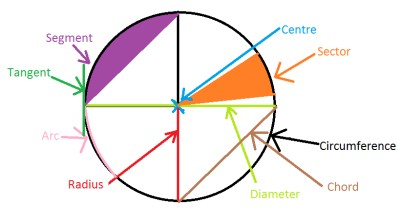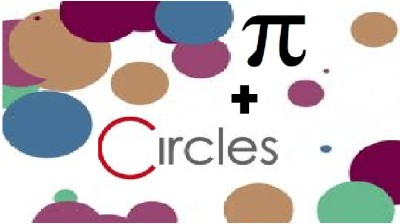List of public pages created with Protopage

# Home

## Plain sticky notes

### Rules when working out equations

1) x + y = y + x An operation is commutative if the order of its operands can be reversed without effecting the result 2) x * y = y * x An operation is commutative if the order of its operands can be reversed without effecting the result 3) x + (y + z) = (x + y) + z An operation is associative if the order of doing multiple operations is not important 4) x * (y * z) = (x * y) * z An operation is associative if the order of doing multiple operations is not important 5) x * (y + z) = (x * y) + (x * z) When there are two operations in this algebra say, + and *, then * is said to be distributive over + 6) x + 0 = x adding 0 does not change result 7) 0 + x = x adding 0 does not change result 8) x * 1 = x multiplying by 1 does not change result 9) 1 * x = x multiplying by 1 does not change result 10) if z = x + y then x = z - y subtract y from both sides of the equation (this is like adding -y) 11) if z = x * y then x = z / y divide both both sides of the equation by y (this is like multiplying by 1/y)

## Calendars

### Calendar

• Sat October 6 - Lunchtime: Buy a new beanie hat
• Sun October 7 - 6pm: Go rollerblading

## Photos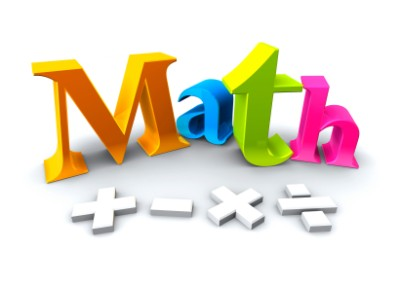# Indices

## Plain sticky notes

### How to....

2^2 means 2x2 2^3 means 2x2x2 2^4 means 2x2x2x2 2^5 means 2x2x2x2x2 2^6 means 2x2x2x2x2x2 2^7 means 2x2x2x2x2x2x2 2^8 means 2x2x2x2x2x2x2x2 2^9 means 2x2x2x2x2x2x2x2x2 2^10 means 2x2x2x2x2x2x2x2x2x2 And so on.... ADDITION AND SUBTRACTION... You can only add and subtract 'like terms'. 3, 4 and 20 are all like terms (because they are all numbers). a, 3a and 200a are all like terms (because they are all multiples of a). a2, 10a2 and -2a2 are all like terms (because they are all multiples of a2) You cannot simplify an expression like 4p + p^2 because 4p and p^2 are not like terms. But you can simplify 3r^2 + 5r^2 + r^2. 3r^2 + 5r^2 + r^2 tells us that we have 'three lots of r^2' + 'five lots of r^2' + 'one lot of r^2' - so in total 'nine lots of r^2', or 9r^2. So, 3r^2 + 5r^2 + r^2 = 9r^2 MULTIPLICATION AND DIVISION... When multiplying you add the indices, and when dividing you subtract the indices. So it follows that: p^3 × p^7 = p^10, and s^5 ÷ s^3 = s^2 For the expression: 4s^3 x 3s^2 The numbers in front of the variables follow the usual rules of multiplication and division, but index numbers follow the rules of indices. So we multiply 4 and 3 and add 3 and 2 4s^3 × 3s^2 = 12s^5

### Sticky note

FIRST RULE... 1^2 x 1^2 = 1^2+2=1^4 (1 to the power anything is 1) It’s is important to leave the answer in indices, notice how the final answer is 1^4 and not the actual value. SECOND RULE... To do the second rule all you have to do is minus the indices. THIRD RULE... For this rule, all you have to do is multiply the indices. FOURTH RULE... The fourth rule means that if the power is a minus number than you have to find the reciprocal and put the number of the power above the denominator. FIFTH RULE... This means that if the power is a fraction, then you need to find the root of the number and root itby the denominator.

### Sticky note

SIXTH RULE... This means that if the power is a fraction, then you need to find the root of the number and root itby the denominator. After that you need to use the numerator as the power.

## Photos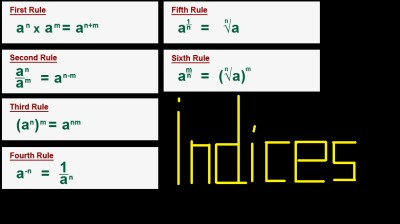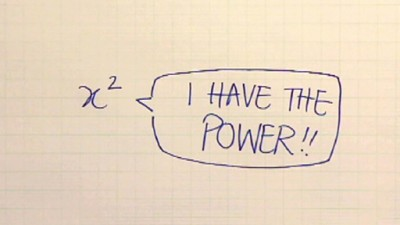# Pi and Circles

## Photos

### The Parts of a circle